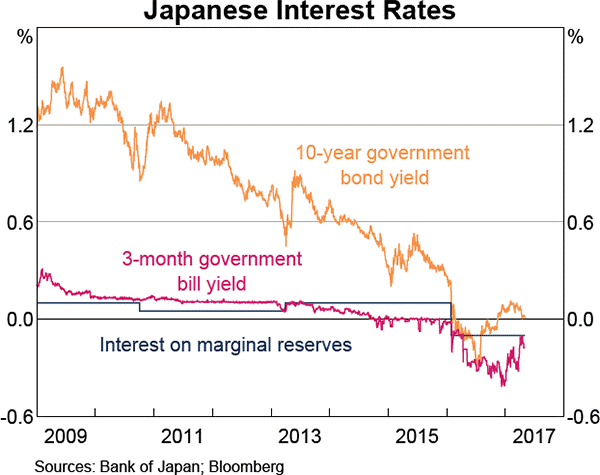# Fight Finance

#### CoursesTagsRandomAllRecentScores

A 180-day Bank Accepted Bill has a face value of $1,000,000. The interest rate is 8% pa and there are 365 days in the year. What is its price now? A 90-day Bank Accepted Bill (BAB) has a face value of$1,000,000. The simple interest rate is 10% pa and there are 365 days in the year. What is its price now?

A 30-day Bank Accepted Bill has a face value of $1,000,000. The interest rate is 8% pa and there are 365 days in the year. What is its price now? A 90-day Bank Accepted Bill has a face value of$1,000,000. The interest rate is 6% pa and there are 365 days in the year. What is its price?

A 60-day Bank Accepted Bill has a face value of $1,000,000. The interest rate is 8% pa and there are 365 days in the year. What is its price now? A 30-day Bank Accepted Bill has a face value of$1,000,000. The interest rate is 2.5% pa and there are 365 days in the year. What is its price now?

On 27/09/13, three month Swiss government bills traded at a yield of -0.2%, given as a simple annual yield. That is, interest rates were negative.

If the face value of one of these 90 day bills is CHF1,000,000 (CHF represents Swiss Francs, the Swiss currency), what is the price of one of these bills?

Refer to the below graph when answering the questions.Which of the following statements is NOT correct?

A 90 day bank bill has a face value of $100,000. Investor A bought the bill when it was first issued at a simple yield to maturity of 3% pa and sold it 20 days later to Investor B who expected to earn a simple yield to maturity of 5% pa. Investor B held it until maturity. Which of the following statements is NOT correct? A bank bill was bought for$99,000 and sold for \$100,000 thirty (30) days later. There are 365 days in the year. Which of the following formulas gives the simple interest rate per annum over those 30 days?

Note: To help you identify which is the correct answer without doing any calculations yourself, the formulas used to calculate the numbers are given.